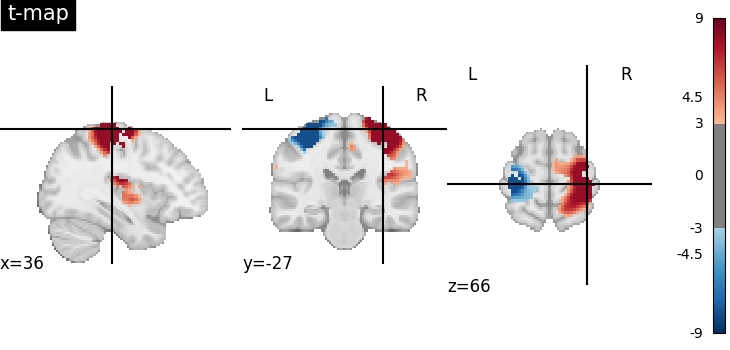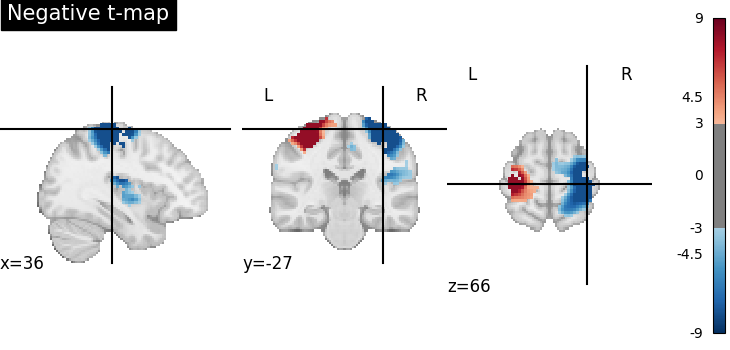# Negating an image with math_img#

The goal of this example is to illustrate the use of the function `nilearn.image.math_img` on T-maps. We compute a negative image by multiplying its voxel values with -1.

```from nilearn import datasets, image, plotting
```

Retrieve the data: a motor contrast map.

```stat_img = datasets.load_sample_motor_activation_image()
```

Multiply voxel values by -1.

```negative_stat_img = image.math_img("-img", img=stat_img)

plotting.plot_stat_map(
stat_img, cut_coords=(36, -27, 66), threshold=3, title="t-map", vmax=9
)
plotting.plot_stat_map(
negative_stat_img,
cut_coords=(36, -27, 66),
threshold=3,
title="Negative t-map",
vmax=9,
)
plotting.show()
```
••Total running time of the script: (0 minutes 4.044 seconds)

Estimated memory usage: 9 MB

Gallery generated by Sphinx-Gallery